﻿ Teaching Chemical Equilibria Using Open Source Software OCTAVE

### Teaching Chemical Equilibria Using Open Source Software OCTAVE

Juan José Baeza-Baeza, Francisco Fernando Pérez-Pla, María Celia García-Álvarez-Coque

World Journal of Chemical Education

## Teaching Chemical Equilibria Using Open Source Software OCTAVE

Juan José Baeza-Baeza1,, Francisco Fernando Pérez-Pla2, María Celia García-Álvarez-Coque1

1Departament de Química Analítica, Universitat de València, c/Dr. Moliner, Burjassot, Spain

2Institut de Ciencia dels Materials (ICMUV), c/Catedrático Beltrán, Paterna, Spain

### Abstract

The use of computational software can greatly facilitate the teaching of chemical equilibria. It allows the treatment of complex problems without the need of simplifications, and the outcome of a very large number of calculations in a short time. Two practical examples of different complexity are proposed in this work to show the application of a general systematic approach for the numerical calculation of multi-equilibria problems, regardless of the number or types of equilibria involved. The method consists in writing down a system of non-linear equations formed by equilibrium constant expressions and the needed number of conservation balances so to match the number of chemical species involved. The exact solution of the system of non-linear equations describing the multiple equilibria is obtained by means of the fsolve tool of the open source software OCTAVE. The approach also allows multiple calculations by automatic changing of the initial conditions. The graphical representation of the concentration of chemical species is also possible as a function of selected variables, such as the initial concentration or the volume of a given reagent.

• Juan José Baeza-Baeza, Francisco Fernando Pérez-Pla, María Celia García-Álvarez-Coque. Teaching Chemical Equilibria Using Open Source Software OCTAVE. World Journal of Chemical Education. Vol. 3, No. 6, 2015, pp 127-133. http://pubs.sciepub.com/wjce/3/6/1
• Baeza-Baeza, Juan José, Francisco Fernando Pérez-Pla, and María Celia García-Álvarez-Coque. "Teaching Chemical Equilibria Using Open Source Software OCTAVE." World Journal of Chemical Education 3.6 (2015): 127-133.
• Baeza-Baeza, J. J. , Pérez-Pla, F. F. , & García-Álvarez-Coque, M. C. (2015). Teaching Chemical Equilibria Using Open Source Software OCTAVE. World Journal of Chemical Education, 3(6), 127-133.
• Baeza-Baeza, Juan José, Francisco Fernando Pérez-Pla, and María Celia García-Álvarez-Coque. "Teaching Chemical Equilibria Using Open Source Software OCTAVE." World Journal of Chemical Education 3, no. 6 (2015): 127-133.

 Import into BibTeX Import into EndNote Import into RefMan Import into RefWorks

12
Prev Next

### 1. Introduction

The calculation of the composition of a system at equilibrium is a basic skill in the formation of Chemistry students. It is also a core subject in Analytical Chemistry and of great importance in other scientific areas, such as Environmental Sciences, Chemical Engineering and Biochemistry [1, 2, 3]. The general approach to calculate equilibrium concentrations consists of solving a system of non-linear equations formed by the equilibrium constant expressions and conservation law balances [4, 5, 6]. However, complex mathematical tools may be required to obtain the correct solution [4, 7, 8]. In order to simplify the problems, different treatments have been applied to different equilibria (acid-base, complexation, precipitation and redox), according to their specific particularities [1, 2]. The chemical equilibrium is considered one of the most difficult topics in the General Chemistry curriculum owing to its abstract character that demands a high capacity of analysis and the expert handling of algebraic concepts . The understanding of the equilibrium nature has been an obstacle for students, leading to difficulties in the correct application of equilibrium concepts, even the relatively simple Le Chatelier's principle . Consequently, teaching of the chemical equilibrium often avoids complex real examples as those involving the combination of reactions of several types. The effect of the electrostatic interaction of ions is also avoided, with the purpose of simplifying the numerical calculation to make it accessible to students.

Today, computational resources are becoming more accessible and reliable. These resources allow the treatment of more complex problems without the need for a deep mathematical background. Teachers have at their disposal software packages, such as MATLAB, OCTAVE, POLYMATH, MATHEMATICA, and EXCEL [4, 11, 12], which enable the study of real examples, focusing the attention on understanding the chemical problem. In this work, we apply the OCTAVE software  to the treatment of the chemical equilibrium. This allows the study of the equilibrium regardless of the number or type of reactions involved. The method consists in writing down a system of non-linear equations formed by equilibrium constant expressions and the needed number of conservation balances so to match the number of chemical species involved. The exact solution of the system of non-linear equations is obtained by means of the fsolve tool of the open source OCTAVE software. This allows solving real problems of high complexity without focusing on the mathematical procedure and keeping the students' attention on the study of the equilibrium problem. The teaching time can be devoted to find the chemical reactions, write the expressions for the equilibrium constants, and establish the chemical species and conservation balances among them. OCTAVE has the advantage of being an open source, which makes it easily available in the web [14, 15]. The OCTAVE language is quite similar to MATLAB  and most programs are easily portable.

The approach completes and improves one previously published for EXCEL , since it makes the arbitrary selection of a group of chemical species to perform the optimization unnecessary. This simplifies handling of the equations. In addition, OCTAVE allows the automatic variation of the initial conditions and the study of the different variables that affect the chemical equilibrium, with the aid of graphs. Two examples are given to illustrate the proposed approach using the OCTAVE language. The first example deals with a mixture of a diprotic acid and a strong base, which forms the basis of many buffer systems in Chemistry and Biochemistry applications . The second example deals with a current issue: the increase of acidity and calcium carbonate solubility in seawater by the rise of atmospheric carbon dioxide (CO2), and its effect on the solubility of marine calcium carbonate [17, 18].

### 2. Methods

The steps of the proposed systematic approach to solve any multi-equilibria problem using OCTAVE is shown in Figure 1.Download asVeiw figureFigures index
Figure 1. Flowchart indicating the steps to solve equilibrium problems using OCTAVE

The balances can be obtained from conservation laws for mass and charge, or considering the stoichiometric ratios between the species involved. Owing to the large difference among the dimensions of the parameters and variables (equilibrium constants can adopt values much greater than unity and the concentrations of the chemical species are usually much lower than unity), the success of the optimization convergence and the reliability of the results requires the inclusion of a normalization step of the equations . This makes the application of numerical methods safer. It also allows the inclusion of all system equations in the minimization process, making the arbitrary selection of a small group of species as main variables unnecessary. An additional strategy that ensures and facilitates the optimization convergence is the use of logarithmic variable transformation, using for instance pX = ‒log X instead of X . This transformation also limits the concentration ranges to positive values and prevents null values. With this approach, the students can fully devote to modeling and understanding the multi-equilibria system, since a proper equation system development requires the correct understanding of the equilibrium problem.

In the next section, two examples are given to illustrate the whole process using the OCTAVE 4.0.0 code . These examples can be applied for practical teaching in the computer lab.

### 3. Results and Discussion

3.1. Calculation of pH and Concentration of All Chemical Species in a Diprotic Acid Buffer System

For the sake of simplicity, we will first describe the approach by solving a relatively simple problem constituted by a mixture of a diprotic weak acid (H2A) and a strong base (NaOH). The initial concentrations are CA and COH, respectively. The steps of the approach are next outlined:

1. Initial species: H2A and NaOH. Initial concentrations: CA = 0.02 M, COH = 0.015 M

2. NaOH is a strong electrolyte: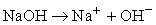(1)

Therefore: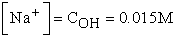(2)

3. The system involves three equilibria (R = 3):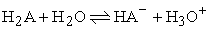(3)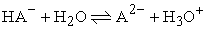(4)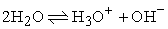(5)

described by the equilibrium constants: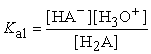(6)(7)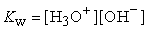(8)

4. In the example, there are five species (S = 5) at equilibrium: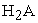,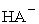,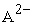,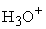, and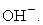5. Therefore, two balances are needed to solve the problem: B = S – R = 5 – 3 = 2

6. The two balances will be the mass and charge balances: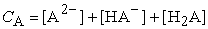(9)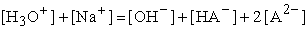(10)

According to Eq. (2), the charge balance becomes: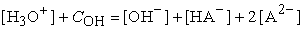(11)

7. The equation system that models the problem is built with Eqs. (6)-(9) and (11). Eqs. (6)-(8) are more conveniently expressed in logarithmic form: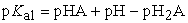(12)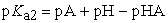(13)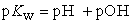(14)

8. The normalized equations are: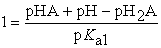(15)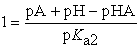(16)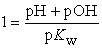(17)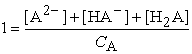(18)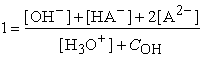(19)

9. The null balance system is: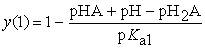(20)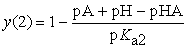(21)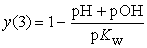(22)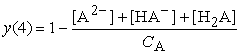(23)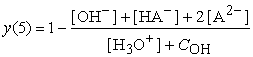(24)

10. The function file with the normalized equations is shown in Box 1 (FILE: diproticbuffer.m)

#### Box 1. OCTAVE code for the computation of the concentration of chemical species involved in multi-equilibria for solutions of the diprotic acid malic acid. Data: C0(1) = 0.02 M (CA), C0(2) = 0.015 M (COH), pK(1) = 3.46 (pKa1), pK(2) = 5.10 (pKa2) and pK(3) = 14 (pKw).a

11. Now, initial values must be assigned to all variables. The following values were chosen: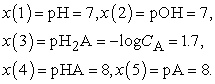12. The main file with the fsolve function is shown in Box 1 (FILE: solvediproticbuffer.m)

13. Taking malic acid as example of diprotic acid and running the solvediproticbuffer.m file, the equilibrium values are: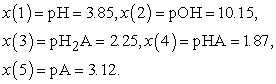Finally, it should be emphasized that the OCTAVE optimization algorithm is powerful enough to allow direct use of Eqs. (6)-(9) and (11) without normalization. However, the proposed procedure ensures the convergence of the minimization process in complex problems, if convenient initial values are given.

The effect of the variation of the initial conditions can be automatically calculated through OCTAVE loop commands. As an example, the effect of the variation in the concentration of the strong base on the concentration of the different species in the diprotic acid system is shown. In Box 2, the OCTAVE file including the loop command is shown (solvespeciesdistribution.m).

#### Box 2. OCTAVE code for the computation of the distribution curve for malic acid. Data: C0(1) = 0.02 M (CHA), C0(2) = 0.00 M (COH), pK(1) = 3.46 (pKa1), pK(2) = 5.10 (pKa2) and pK(3) = 14 (pKw).aDownload asVeiw figureFigures index
Figure 2. Distribution of chemical species obtained with OCTAVE for a diprotic acid system by changing the concentration of strong base

Figure 2 shows the variation of the concentrations of the chemical species by changing the concentration of strong base. This figure is easily obtained by means of the plot command. In order to assure convergence, the values obtained in a previous iteration should be adopted as initial values for the next iteration.

Other graphs, such as distribution diagrams, ‒log C versus pH diagrams, or titrations curves, can be easily obtained with a small manipulation of the software code. Next, the example is slightly modified to show how the pH changes with the volume (mL) of NaOH solution along a titration. In this case, the volumes play an important role and the initial concentrations of titrand and titrator are fixed. It should be considered that the concentrations of both acid and base change due to dilution along the titration. The code is presented in Box 3.

#### Box 3. OCTAVE code for the computation of a titration curve for malic acid. Data: V0 = 20 mL, CI(1) = 0.05 M (CHA), CI(2) = 0.05 M (COH), pK(1) = 3.46 (pKa1), pK(2) = 5.10 (pKa2) and pK(3) = 14 (pKw).a

Figure 3 shows the variation of the pH with the added volume of strong base as calculated by the OCTAVE code given in Box 3.Download asVeiw figureFigures index
Figure 3. Titration curve for 0.05 M malic acid with 0.05 M sodium hydroxide

For malic acid, a single steep rise is observed in the titration curve, since the acid-base constants are too close to each other. Changing the values of the constants, two neat jumps may be observed, as is the case of oxalic acid.

3.2. Rising of the Acidity and Calcium Carbonate Solubility in the Ocean by the Increase of Atmospheric CO2

It is widely recognized that the rising level of atmospheric CO2 is causing Earth’s global warming. Recently, another problem caused by the massive absorption of CO2 by the oceans has been studied: The pH of seawater has remained unchanged for millions of years. Therefore, an acidity increase could produce a notable influence on several organisms that use carbonate ion to build their skeletons . In this example, the effect of the rising level of atmospheric CO2 is studied as a multi-equilibria problem . The steps to follow are shown below:

1. The initial species are the atmospheric CO2 with a given pressure (in this case PCO2 is varied from 10‒6 to 10‒3 atm), and a sufficient stock of calcium carbonate in the ocean to ensure compliance of the solubility product.

2. In this example there are no strong electrolytes.

3. Five equilibria are met (R = 5):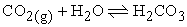(25)(26)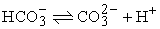(27)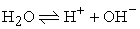(28)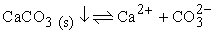(29)

described by the equilibrium constants: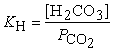(30)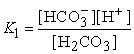(31)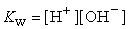(33)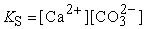(34)

H2CO3 accounts for the dissolved CO2, which consists mostly of the hydrated species.

4. There are six species at equilibrium (S = 6):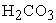,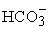,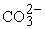, H+, OH and Ca2+.

5. Only one balance is therefore required: B = S  – R = 6 ‒ 5 = 1

6. It will be the charge balance: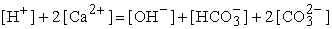(35)

7. The final equation system is expressed using the logarithms of the equilibrium expressions in Eqs. (30) to (34):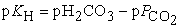(36)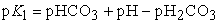(37)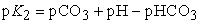(38)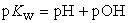(39)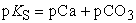(40)

together with the charge balance (Eq. (35)).

8. The normalized equations are: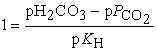(41)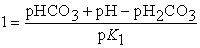(42)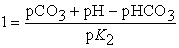(43)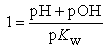(44)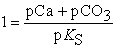(45)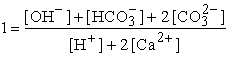(46)

9. The final null equations are: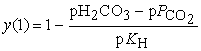(47)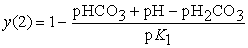(48)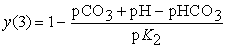(49)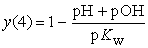(50)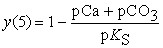(51)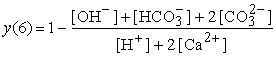(52)

10. The function file is shown in Box 4 (carbonateequilibria.m)

11. The CO2 pressure now ranges from 10‒6 to 10‒3 atm (1 to 1000 ppmv).

12. The main file with the loop function to calculate the concentrations of the chemical species is also shown in Box 4.

#### Box 4. OCTAVE code for the computation of carbonate equilibria in seawater. Data: P0(1) = p (atmospheric CO2 in atm from 0.000001 to 0.001), pK(1) = 5.84 (pKa1), pK(2) = 8.91 (pKa2), pK(3) = 13.21 (pKw), pK(4) = 6.37 (pKs) and pK(5) = 1.63 (pKH).a,b

13. The results are depicted in Figure 4.Download asVeiw figureFigures index
Figure 4. Effect of increasing atmospheric CO2 concentration on carbonate solubility and pH in sea water

Figure 4 allows studying the effect of an increased level of CO2 in the atmosphere on the acidity of seawater. It can be seen that the effect is greater the lower the concentration of CO2, because at the lowest values seawater is basic due to the solubility of carbonates. By adsorption of CO2, a carbonic-bicarbonate buffer is formed, giving rise to an almost linear variation of both pH and carbonate solubility.

### 4. Conclusions

The open source software OCTAVE allows the rigorous resolution of any equilibrium problem, without the need for deep mathematical knowledge. Only simple algebra and logarithm basics are needed to build and manipulate the system of non-linear equations formed by the equilibrium constants, and charge and mass balances. Now students can give full attention to understand the chemical problem, and devote all their time to find out the species involved, the equilibria reactions, the equilibrium constant expressions, and the conservation balances. There is no restriction in the type of reactions that can be included in the problem. Only knowledge of the equilibrium constants is required. Students should control if each reaction included is still valid at the final equilibrium conditions. For instance, when an ionic solid is fully dissolved, its solubility product should be removed.

For those students who do not like using spreadsheets for calculations, this software provides a more flexible and powerful alternative, which would make it suitable for many types of problems. OCTAVE requires the students personally manipulate the programming code to explicitly write the equations that govern the chemical system, making their own programs. Finally, students will also gain experience with a method for solving simultaneous equations using OCTAVE.

The proposed methodology is complementary to the traditional one for solving equilibrium problems. In the next future, software as OCTAVE and MATLAB, or similar, will be commonly used in the computer lab to address complex examples, whose exact resolution cannot be carried out with commonly used simplified equations. For first year students of General Chemistry and second year students of Analytical Chemistry, good results have been obtained by providing built programs (such as Box 2 and Box 3). Students would only build the equations that describe the equilibria. Changes may be made in the initial values of the concentrations and equilibrium constants, in order to quickly observe their effect on the equilibrium. For example, titration curves for diprotic acids with different pK values could be built to see their effect on the two jumps observed at the equivalence points.

With the proposed approach, students gain the confidence that their equilibrium problem will be solved without mistakes, even with complex real examples. The approach gives the students tools to cope with more numerous and complex equilibrium problems, after understanding them.

### References

  Burgot, J. L. Ionic Equilibria in Analytical Chemistry, Springer, New York, 2012.In article View Article  PubMed  Skoog, D. A., West, D. M., Holler, F. J., Crouch, S. R., Fundamentals of Analytical Chemistry, Cengage Learning, Boston, MA, 2014.In article  Stumm, W., Morgan, J. J., Aquatic Chemistry: Chemical Equilibria and Rates in Natural Waters, Wiley, New York, 1995.In article PubMed  Baeza-Baeza, J. J., García-Alvarez-Coque, M. C., “Systematic Approach to Calculate the Concentration of Chemical Species in Multi-Equilibrium Problems”, J. Chem. Educ., 88, 169-173, 2011.In article View Article  Baeza-Baeza, J. J., García-Alvarez-Coque, M. C., “Extent of Reaction Balances: A Convenient Tool to Study Chemical Equilibria”, World J. Chem. Educ., 2, 54-58, 2014.In article  Salvatore, M. M., Guida, M., Salvatore F., “pH and Acid-Base Equilibrium Calculations via a Matrix Representation of Solutions of Acids and/or Bases”, World J. Chem. Educ., 3, 93-110, 2015.In article  Wall, T. W., Greening, D., Woolsey, R. E. D., “Solving Complex Chemical Equilibria Using a Geometric-Programming Based Technique”, Oper. Res., 34, 345-355, 1986.In article View Article  Paz-García, J. M., Johannesson, B., Ottosen, L. M., Ribeiro, A. B., Rodríguez-Maroto, J. M., “Computing Multi-Species Chemical Equilibrium with an Algorithm based on the Reaction Extents”, Comput. Chem. Eng., 58, 135-143, 2013.In article View Article  Van Driel, J. H., De Vos, W., Verloop, N., Dekkers, H., “Developing Secondary Students’ Conceptions of Chemical Reactions: the Introduction of Chemical Equilibrium”, Int. J. Sci. Educ., 20, 379-392, 1998.In article View Article  Quilez J., “Changes in Concentration and in Partial Pressure in Chemical Equilibria: Students' and Teachers' Misunderstandings”, Chem. Educ. Res. Pract., 5, 281-300, 2004.In article View Article  Glaser, R. E., Delarosa, M. A., Salau, A. O., Chicone, C., “Dynamical Approach to Multi-Equilibria Problems for Mixtures of Acids and their Conjugated Bases”, J. Chem. Educ., 91, 1009-1016, 2014.In article View Article  Cutlip, M. B., Shacham, M., Problem Solving in Chemical and Biochemical Engineering with POLYMATH, EXCEL, and MATLAB, Prentice Hall, Upper Saddle River, NJ, 2008.In article  Eaton, J. W., “GNU Octave and Reproducible Research”, J. Process Control, 22, 1433-1438, 2012.In article View Article  Available: http://www.gnu.org/software/octave/ [Accessed Oct. 23, 2015].In article  Quarteroni, A., Saleri, F., Gervasio, P., Scientific Computing with MATLAB and OCTAVE, 4th Ed., Springer, Heidelberg, 2014.In article View Article  Attaway, S., MATLAB. A Practical Introduction to Programming and Problem Solving, Elsevier, Amsterdam, 2013.In article PubMed  Doney, S. C., Fabry, V. J., Feely, R. A., Kleypas, J. A., “Ocean Acidification: The Other CO2 Problem”, Annu. Rev. Mar. Sci., 1, 169-92, 2009.In article View Article  PubMed  Millero, F. J., “Thermodynamics of the carbon dioxide system in the oceans”, Geochim. Cosmochim. Acta, 59, 661-677, 1995.In article View Article